# Advanced Geometry : How to find the volume of a tetrahedron

## Example Questions

2 Next →

### Example Question #11 : Volume

How is the volume of a regular tetrahedron effected when the length of each edge is doubled?

It is 4 times greater.

It is 8 times greater.

It increases by 50%.

It is doubled.

It cannot be determined by the information given.

It is 8 times greater.

Explanation:

The volume of a regular tetrahedron is found with the formula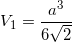whereis the length of the edges.

The volume of the same tetrahedron when the length of the edges are doubled would be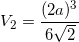.

Therefore,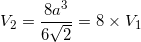### Example Question #12 : Volume

What is the volume of a regular tetrahedron with edges of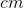?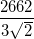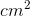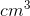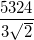None of the above.Explanation:

The volume of a tetrahedron is found with the formula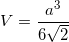whereis the length of the edges.

When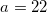,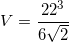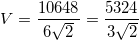And, of course, volume should be in cubic measurements!

### Example Question #13 : Volume

Find the volume of a regular tetrahedron if one of its edges is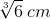long.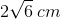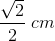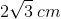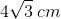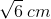Explanation:

Write the volume equation for a tetrahedron.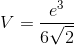In this formula,stands for the tetrahedron's volume and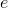stands for the length of one of its edges.

Substitute the given edge length and solve.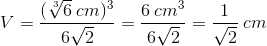Rationalize the denominator.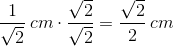### Example Question #14 : Volume

Find the volume of a tetrahedron if the side length is.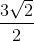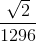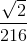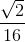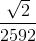Explanation:

Write the equation to find the volume of a tetrahedron.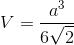Substitute the side length and solve for the volume.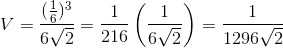Rationalize the denominator.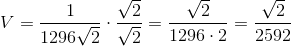### Example Question #21 : 3 Dimensional Geometry

What is the volume of a regular tetrahedron with an edge length of 6?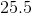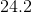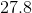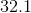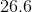Explanation:

The volume of a tetrahedron can be solved for by using the equation:whereis the measurement of the edge of the tetrahedron.

This problem can be quickly solved by substituting 6 in for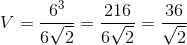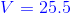### Example Question #22 : 3 Dimensional Geometry

What is the volume of the tetrahedron shown below?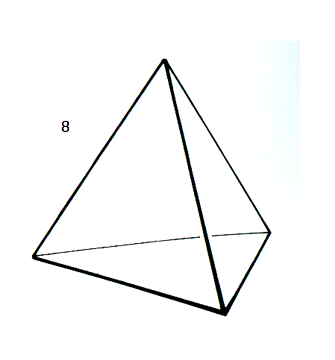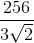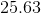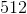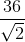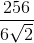Explanation:

The volume of a tetrahedron is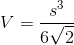.

This tetrahedron has a side with a length of 8.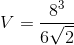, which becomes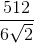.

You can reduce that answer further, so that it becomes2 Next →